# 4.3 Mesh modification modules

An alphabetic list of the mesh modification modules is given below (we start with the utility module INITI):

INITI:

Aim:
Initializes the variables used by all modules in the MODULEF library, in particular initialization of dynamic storage. Calling this utility is thus indispensable in all the main programs written by the user.
Calling the utility:

CALL INITI (M,LM,IMPRE,NNN)

where

• M: the super array
• LM: the array length in number of words
• IMPRE: the output parameter for execution (0 no output, ...., 10 maximum output)
• NNN: the print parameter for arrays (0 no output, ... 3 maximum output), i.e., each operation concerning the dynamic storage of an array in the super array M is indicated.

Data:
This subroutine reads a TITLE in free format.
Remark:
The subroutine INITIS has the same arguments as INITI and fulfills the same role, but does not require a title.

I.D.S. NOPO
O.D.S. NOPO

Aim:
Defines the element nodes when they differ from the element vertices. This module is used to add nodes on the edges, faces, or to the element interiors. It furthermore determines whether the vertices coincide with the nodes.

From a P1 (nodes = vertices) type starting mesh , the module generates the nodes and assigns a number to each one (the exact positions of the nodes are calculated by the interpolation module). This operation is performed in order to allow for an optimal numbering of the nodes.

For compatibility reasons, the number of nodes in a triangle, or on a triangular face, must equal 1 or a multiple of 3. Similarly, inside a quadrilateral, or on a quadrilateral face, the number of nodes must be 1 or a multiple of 4.

Calling the module:
By keyword ADPO of APNOPO (2D case) or
 APNOP3 (3D case), or by a classical call:

Dimension array M (in the blank common), call INITI

. NFNOPE,NINOPE,NFNOPS,NINOPS)

where

• M: the super array
• ISEG: the number of nodes on the edges (end-nodes excluded)
• ISET: the number of internal nodes in each triangle, or triangular face
• ISEQ: the number of internal nodes in each quadrilateral, or quadrilateral face
• ISETE: the number of internal nodes in each tetrahedron
• ISEPE: the number of internal nodes in each pentahedron
• ISEHE: the number of internal nodes in each hexahedron
• NOESOM: 1 if the vertices and nodes coincide, 0 if not
• NF(NI)NOPE: the support number of the I.D.S. NOPO and its level
• NF(NI)NOPS: the same for the O.D.S. NOPO

AFFNOP:
I.D.S. NOPO
O.D.S. NOPO

Aim:
Refine a P1 type mesh  around vertices specified. This module can perform several successive refinements  around the same vertex, by repeating it as many times as desired in the list of vertices serving as input data.

The method is only suitable for the two-dimensional case.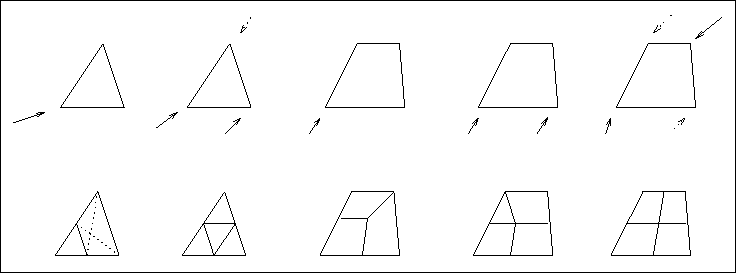Figure 4.4: Refinement around vertices

Many situations are possible depending on the type of element, for which at least one point is given in the list of points to treat, and the position of the vertices under consideration (see figure 4.4).

Calling the module:
By keyword AFFL of APNOPO (2D case), or by a classical call:

Dimension array M (in the blank common) and arrays NSAT and
NNFRON, declare the logical FONINT, declare the external FFRONT, call INITI

. LSAT,NSAT,NBFRON,NNFRON,FONINT,FFRONT)

where

• M: the super array
• NF(NI)NOPE: the support number of the I.D.S. NOPO and its level
• NF(NI)NOPS: the same for the O.D.S. NOPO
• NQUAD: 1 if the creation of quadrilateral are authorized when treating a triangle, 1 if not
• LSAT: the number of vertices to consider (counted as many times as required)
• NSAT(.): an integer array (dimension LSAT) containing the numbers of the vertices to consider (appearing as many times as necessary)
• NBFRON: the number of curved boundaries
• NNFRON(.): an integer array (dimension NBFRON) containing the reference numbers of these boundaries
• FONINT: a logical initialized to .FALSE.
• FFRONT: the name of the function for possible projections on the curved boundaries

Remark:
Function FFRONT is defined in chapter 1.

affini.psfig:aff2Initial meshaffout.psfig:aff21After refinement

AIGUNO:
I.D.S. NOPO
O.D.S. NOPO

Aim:
Remove the obtuse angles  from a two-dimensional mesh  composed of triangles.
Calling the module:
Keyword AIGU of APNOPO, or a classical call:

Dimension array M (in the blank common), call INITI

CALL AIGUNO (M,NOPTION,NITER,NFNOPE,NINOPE,
. NFNOPS,NINOPS)

where

• M: the super array
• NOPTION: processing option:
• 1: execute the module
• 0: the module is not executed; the triangles with obtuse angles are marked by setting the sub-domain number to the value -10 (for visualization purposes)
• NITER: the number of iterations to perform (2,3,...)
• NF(NI)NOPE: the support number of the I.D.S. NOPO and its level
• NF(NI)NOPS: the same for the O.D.S. NOPO

Remark:
The following information is stored in an associated integer array, called IFRO, of length NP+1, where NP is the number of vertices in the mesh:
• IFRO(1) = MAX, where MAX is the maximum number of neighbors of a vertex
• IFRO(I+1) = 0 if vertex I is internal, k if not, where k is the number of the boundary on which vertex I is found.

AKHHAT:
I.D.S. NOPO
O.D.S. NOPO

Aim:
Renumber  the nodes, or nodes and elements, of a mesh. The Akhras and Gouri Dhatt method  is used.
Calling the module:
Classical call:

Dimension array M (in the blank common), call INITI

CALL AKHHAT (M,NOCODE,NITER,NFNOPE,NINOPE,NFNOPS,
. NINOPS)

where

• M: the super array
• NOCODE: renumbering option: 0 only the nodes, 1 the nodes and elements
• NITER: the number of iterations to perform (1 or 2 are generally enough)
• NF(NI)NOPE: the support number of the I.D.S. NOPO and its level
• NF(NI)NOPS: the same for the O.D.S. NOPO

Remark:

CLNO** :

Aim:
The utilities CLNO5C , CLNOIA , CLNOIN  and CLNOPO  are used to read array NOP5 of a D.S. NOPO and extract certain information from it without getting tangled up with the management of the element addresses contained in this array.

CLNO5C:
This utility is used to obtain NCGE, NDSDE, NNO and NONO(.), the number and list of nodes of the element under consideration, NPO and NOPOI(.), the number and list of its points as well as their coordinates by passing the input parameters: NDIM, NCOPNP, array NOP5, the address IA of an element, and array NOP4.

Dimension the arrays NONO, NOPOI, COORP and call CLNO5C as follows:

CALL CLNO5C (NDIM,NCOPNP,NOP5,IA,NCGE,NDSDE,NNO,
. NONO,NPO,NOPOI,NOP4,COORP)

where

• NDIM: the space dimension
• NCOPNP: the coincidence code nodes-points
• NOP5: array NOP5 of the D.S. to read
• IA [I]: the address of the element under consideration
• NOP4: array NOP4 (the coordinates) of the D.S.
• IA [O]: the address of the next element
• NCGE [O]: the geometric code of the element
• NDSDE [O]: its sub-domain number
• NNO and NONO(.) [O]: the number and list of nodes
• NPO and NOPOI(.) [O]: the number and list of points
• COORP(.) [O]: the coordinates of the above points (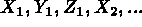)

CLNOIA:
This utility is used to obtain NCGE, NPO and NOPOI(.), the number and list of points of the element, as well as IAS, IAR, IAF, the addresses in NOP5 of the point, edge and face references of this element, by passing the input parameters: NCOPNP, array NOP5 and the address IA of an element.

Dimension array NOPOI and call CLNOIA as follows:

CALL CLNOIA (NCOPNP,NOP5,IA,NCGE,IAS,IAR,IAF,NPO,NOPOI)

where

• NCOPNP: the coincidence code nodes-points
• NOP5: array NOP5 of the D.S. to read
• IA [I]: the address of the element under consideration
• IA [O]: the address of the next element
• NCGE [O]: the geometric code of the element
• IAS [O]: the address in NOP5 of the reference of the first point of the element, if no point is referenced, IAS = 0
• IAR [O]: the address in NOP5 of the reference of the first edge of the element, if no edge is referenced, IAR = 0
• IAF [O]: the same for the element faces, if no face is referenced,
IAF = 0
• NPO and NOPOI(.) [O]: the number and list of points

CLNOIN:

Similar to CLNOIA, this utility is used to obtain NNO and NONO(.), the number and list of the nodes of the element.

Dimension array NONO and call CLNOIN as follows:

CALL CLNOIN (NCOPNP,NOP5,IA,NCGE,IAS,IAR,IAF,NPO,NNO,
. NONO)

where

• NCOPNP: the coincidence code nodes-points
• NOP5: array NOP5 of the D.S. to read
• IA [I]: the address of the element under consideration
• IA [O]: the address of the next element
• NCGE [O]: the geometric code of the element
• IAS [O]: the address in NOP5 of the reference of the first point of the element, if no point is referenced, IAS = 0
• IAR [O]: the address in NOP5 of the reference of the first edge of the element, if no edge is referenced, IAR = 0
• IAF [O]: the same for the element faces, if no face is referenced,
IAF = 0
• NPO [O]: the number of points
• NNO and NONO(.) [O]: the number and list of nodes

CLNOPO:
This utility is similar to CLNO5C, but references the super array M instead of array NOP5 directly. This module is used to obtain NCGE, NDSDE, NNO and NONO(.), the number and list of nodes of the element under consideration, NPO and NOPOI(.), the number and list of its points, their coordinates and the references of the vertices, edges and faces of this element.

Dimension arrays NONO, NOPOI, NREF and COORP, and call CLNOPO as follows:

CALL CLNOPO (M,M,IA2,IA4,IA,NCGE,NDSDE,NNO,NONO,
. NPO,NOPOI,COORP,NREF)

where

• M: the super array
• IA [I]: the address in NOP5 of the element under consideration
• IA2 and IA4 [I]: the addresses of arrays NOP2 and NOP4 of the D.S.
• IA [O]: the address of the next element
• NCGE [O]: the geometric code of the element
• NDSDE [O]: its sub-domain number
• NNO and NONO(.) [O]: the number and list of nodes
• NPO and NOPOI(.) [O]: the number and list of points
• COORP(.) [O]: the coordinates of the points above ()
• NREF(.) [O]: the vertex references, followed by the edge and, lastly, face references

Remarks:

The order of array NREF is the inverse order of that of array NOP5.

NREF is completed systematically, even if the references are all zero.

Generally, and for all the CLNO** utilities, the arrays are declared by over-estimating their length.

An example:

```      SUBROUTINE REFNOP(M,XM,DM,NFNOPE,NINOPE,NREFE,INDIC,LINDIC)
C +++++++++++++++++++++++++++++++++++++++++++++++++++++++++++++++++++
.
C
C     OVER-ESTIMATE THE NUMBER OF NODES (I.E. OF POINTS) BY 20 ...
C
NDIM  = M(IANOP2)
NOE23 = NDIM * 20
NOE2  = 2 * 20
IAPOI = IANOE + 20
LREF  = 26
C
LINDIC = 0
IANOP5 = M( ICNOPO + 17 )
IA     = IANOP5
DO 10 J=1,NE
CALL CLNOPO(M,M,IANOP2,IANOP4,IA,NCGE,NDSDE,NNO,M(IANOE),
+               NPO,M(IAPOI),XM(IACOO),M(IAREF))
LONG  = NTOT(NCGE)
ITEST = 0
DO 1 K=1,LONG
IF ( M(IAREF+K-1) .NE. 0 ) ITEST = 1
1    CONTINUE
IF ( ITEST .EQ. 0 ) GO TO 10
IDEB = LONG - NFAC(NCGE) + 1
IF ( NDIM .EQ. 2) IDEB = LONG - NARE(NCGE) + 1
DO 2 K=IDEB,LONG
IF ( M(IAREF+K-1) .NE. NREFE ) GO TO 2
LINDIC = LINDIC + 1
INDIC(LINDIC) = J
GO TO 3
2    CONTINUE
3    CONTINUE
10 CONTINUE
.
END
```

COULE1:
I.D.S. NOPO
O.D.S NOPO

Aim:
Renumber the elements  of a mesh to form disjoint sets of elements. The method corresponds to coloring the elements carefully so that 2 neighboring elements do not have the same color .

The aim of this procedure is to allow for vector and/or parallel computing, for example at the element matrix calculation level.

Calling the module:
By keyword COUL of APNOPO (2D case) or APNOP3 (3D case), or a call via preprocessor COULXX, or a classical call:

Dimension array M (in the blank common), declare the integer array COURB of length NLC, call INITI

CALL COULE1 (M,M,NFNOPE,NINOPE,NFNOPS,NINOPS,
. LVECT,NOMCOU,COURB,NLC)

where

• M: the super array
• NF(NI)NOPE: the support number of the I.D.S. NOPO and its level
• NF(NI)NOPS: the same for the O.D.S. NOPO
• LVECT: maximum number of elements per color
• NOMCOU: name of the associated array
• COURB: the reference numbers of the curved lines
• NLC: the number of curved lines

Remark:
The associated array contains a pointer to the colors, indicating that elements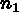to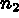have color 1, etc.

DEFNOP:
I.D.S. NOPO and B
O.D.S. NOPO

Aim:
Construct the  deformation of a mesh (I.D.S. NOPO) by adding the displacement values (I.D.S. B) to the coordinates of the element vertices. It corresponds therefore to the postprocessing of a 2D or 3D elasticity problem . The result is a D.S. NOPO that can be visualized.

The method works for Lagrange type elements or elements for which the first 2 (in 2D) or 3 (in 3D) d.o.f. correspond to the displacements.

Calling the module:
Via preprocessor DEFNXX or a classical call:

Dimension array M (in the blank common), call INITI

CALL DEFNOP (M,M,M,NFNOPE,NINOPE,NFB,NIB,RAPORT,NO,
. NFNOPS,NINOPS,NFNDL1,NINDL1)

where

• M: the super array
• NF(NI)NOPE: the support number of the I.D.S. NOPO and its level
• NF(NI)B: the support number of the I.D.S. B and its level
• RAPORT: the displacement amplification factor
• NO: the number of the load case to process
• NF(NI)NOPS: the same as above for the O.D.S. NOPO
• NF(NI)NDL1: the support number of the I.D.S. NDLI and its level, D.S. necessary if ND = 0, if not set NFNDL1 to 0.

DILNOP:
I.D.S. NOPO
O.D.S. NOPO

Aim:
Construct the  deformation of a mesh (I.D.S. NOPO) by anisotropic dilation .

The method works for P1 Lagrange type elements.

Calling the module:
By keyword DILA of APNOPO (2D) or APNOP3 (3D), or a classical call:.

Dimension array M (in the blank common), declare the integer arrays NNF and NNDSD of length 2 (if not utilized) or 2 LNNF and 2 LNDSD (see below), call INITI

CALL DILNOP (M,NFNOPE,NINOPE,NFNOPS,NINOPS,A,B,C,X0,
. Y0,Z0,LNNF,NNF,LNDSD,NNDSD)

where

• M: the super array
• NF(NI)NOPE: the support number of the I.D.S. NOPO and its level
• NF(NI)NOPS: the same for the O.D.S. NOPO
• A,B,C: the dilation factors in the OX,OY,OZ directions
• X0,Y0,Z0: the coordinates of an invariant point
• LNNF: the number of references to modify
• NNF: the reference correspondence array:
If= NNF(1,j) and= NNF(2,j) then the items with number(j-th number to modify) will have the numberin the O.D.S.
• LNDSD: the number of sub-domain numbers to modify
• NNF: the array indicating the correspondence between these numbers (same logic as above)

DTRI3D:
I.D.S. NOPO
O.D.S. NOPO
Aim:
Split the elements  of a 3D mesh composed of tetrahedra, pentahedra and hexahedra into tetrahedra, without adding points .

The I.D.S. is a P1 Lagrange type data structure.

Calling the module:
Keyword TETR of APNOP3 (3D), or a classical call:

Dimension array M (in the blank common), call INITI

CALL DTRI3D (M,NFNOPE,NINOPE,NFNOPS,NINOPS,IOPT)

where

• M: the super array
• NF(NI)NOPE: the support number of the I.D.S. NOPO and its level
• NF(NI)NOPS: the same for the O.D.S. NOPO
• IOPT: splitting option: 5 if the hexahedra are split preferentially into 5 tetrahedra, different from 5 if not

ELIMNO:
I.D.S. NOPO
O.D.S. NOPO
Aim:
Eliminate one or several elements  from a   NOPO type I.D.S. and create the corresponding NOPO type O.D.S.

Calling the module:
Via preprocessor ELIMXX, or a classical call:

Dimension array M (in the blank common), declare array LISTE and call INITI

CALL ELIMNO (M,NFNOPE,NINOPE,NBRE,LISTE,NFNOPS,
. NINOPS,IAPOI)

where

• M: the super array
• NF(NI)NOPE: the support number of the I.D.S. NOPO and its level
• NBRE: the number of elements to remove from the mesh
• LISTE: the array containing the list of its elements
• NF(NI)NOPS: the support number of the O.D.S. NOPO and its level
• IAPOI [O]: the address in M of the array containing the correspondence between the old and new vertex numbers

GEONOP:
I.D.S. GEOM
O.D.S. NOPO

Aim:
Convert  a   GEOM type I.D.S. into a NOPO type O.D.S.

Calling the module:
Via preprocessor GEONXX, or a classical call:

Dimension array M (in the blank common), call INITI

CALL GEONOP (M,NFGEOM,NIGEOM,NFNOPS,NINOPS)

where

• M: the super array
• NF(NI)GEOM: the support number of the I.D.S. GEOM and its level
• NF(NI)NOPS: the same for the O.D.S. NOPO

GIBBS:
I.D.S. NOPO
O.D.S. NOPO
Aim:
Renumber   the nodes, or the nodes and elements, of a mesh. The Gibbs method   is employed.

Calling the module:
Keyword RENC of APNOPO (2D case), or APNOP3 (3D case) to renumber the nodes of a mesh, or keyword RENE to renumber the nodes and elements, or a call via preprocessor GIBBXX, or lastly a classical call:

Dimension array M (in the blank common), call INITI

CALL GIBBS (M,NOCODE,NFNOPE,NINOPE,NFNOPS,NINOPS,
. NOMTAB,LONTAB,IANOUV)

where

• M: the super array
• NOCODE: renumbering option: 0 only the nodes, 1 the nodes and elements
• NF(NI)NOPE: the support number of the I.D.S. NOPO and its level
• NF(NI)NOPS: the same for the O.D.S. NOPO
• NOMTAB [O]: name of the correspondence array (see the remark below)
• LONTAB [O]: its length
• IANOUV [O]: its address in M

Remarks:

The array called NOMTAB, of length LONTAB and address IANOUV, is used to determine the correspondence between the new and old node numbers:

M(IANOUV+I-1) = new number of old node I

An example:
example n� 1 of chapter 5.

``` &&&&&&&&&&&&&&&&&&&&&&&&&&&&&&&&&&&&&&&&&&&&&&&&&&&&&&&&&&&&&&&&&&&&&&&&&&&&&&
MODULE GIBBS : PART_OVEN
&&&&&&&&&&&&&&&&&&&&&&&&&&&&&&&&&&&&&&&&&&&&&&&&&&&&&&&&&&&&&&&&&&&&&&&&&&&&&&
&&&&&&&&&&&&&&&&&&&&&&&&&&&&&&&&&&&&&&&&&&&&&&&&&&&&&&&&&&&&&&&&&&&&&&&&&&&&&&
MODULE RENUM1 : PART_OVEN
&&&&&&&&&&&&&&&&&&&&&&&&&&&&&&&&&&&&&&&&&&&&&&&&&&&&&&&&&&&&&&&&&&&&&&&&&&&&&&

PERMUTATION OF THE COORDINATES
------------------------------

RENUMBERING OF THE NODES
-------------------------

NUMBER OF NODES :       166

------------------------------------------------------------------
I CARACTERISTICS   I BEFORE RENUMBERING   I AFTER RENUMBERING    I
------------------------------------------------------------------
I BAND WIDTH       I              120     I               16     I
------------------------------------------------------------------
I TOTAL PROFILE    I             4247     I             2034     I
I MEAN PROFILE     I               25.584 I               12.253 I
------------------------------------------------------------------
&&&&&&&&&&&&&&&&&&&&&&&&&&&&&&&&&&&&&&&&&&&&&&&&&&&&&&&&&&&&&&&&&&&&&&&&&&&&&&
END OF MODULE RENUM1
&&&&&&&&&&&&&&&&&&&&&&&&&&&&&&&&&&&&&&&&&&&&&&&&&&&&&&&&&&&&&&&&&&&&&&&&&&&&&&
&&&&&&&&&&&&&&&&&&&&&&&&&&&&&&&&&&&&&&&&&&&&&&&&&&&&&&&&&&&&&&&&&&&&&&&&&&&&&&
END OF MODULE GIBBS
&&&&&&&&&&&&&&&&&&&&&&&&&&&&&&&&&&&&&&&&&&&&&&&&&&&&&&&&&&&&&&&&&&&&&&&&&&&&&&
```

IMGEOM:
I.D.S. GEOM

Aim:
Print the   contents   of a GEOM  type I.D.S.
Calling the module:
Via preprocessor IMAGXX, or a classical call:

Dimension array M (in the blank common), call INITI

CALL IMGEOM (M,NFGEOM,NIGEOM,IMPRE)

where

• M: the super array
• NF(NI)GEOM: the support number of the I.D.S. GEOM and its level
• IMPRE: the output parameter from 0 to 10

IMNOPO:
I.D.S NOPO

Aim:
Print the  contents  of a NOPO type D.S.
Calling the module:
Keyword IMPR of APNOPO or APNOP3, or via preprocessor IMAGXX, or a classical call:

Dimension array M ( in the blank common), call INITI

CALL IMNOPO (M,NFNOPO,NINOPO,IMPRE)

where

• M: the super array
• NF(NI)NOPO: the support number of the I.D.S. NOPO and its level
• IMPRE: the output parameter from 0 to 10

A complete description of the NOPO data structure will now be given.

Constructed along the same principles as the other data structures, it is composed of six arrays of predefined organization.

Array NOP0:
General information.

This integer array consists of 32 variables. It contains a general description of the job (title, date, name), the D.S. NOPO (type, level, etc.), and indicates the presence of the array NOP1.

• 1..20 TITLE, the job title in 20 words of 4 characters,
• 23.28 NOMCRE, the name of the creator in 6 words of 4 characters,
• 29 'NOPO', the structure type,
• 30 NIVEAU, the D.S. level parameter,
• 31 ETAT, a reserved parameter,
• 32 NTACM, the number supplementary arrays associated with the structure (they are described in array NOP1).

Array NOP1:
Descriptor of the supplementary arrays.

This integer array exists if NTACM (see NOP0) is non-zero. It contains 22 variables per array. In general, there are no arrays associated except for certain applications where it is necessary to conserve the values that do not enter in its standard definition in the data structure.

If the array NOP1 exist, it contains sequentially:

Loop I from 1 to NTACM

• name of array I in 4 characters,
• address of array I in super array M,
• number of words in this array,
• array type (1 = integer, 2 = real simple precision, 4 = character, 5 = real double precision, etc.)
• comment on the contents of array I in 18 words of 4 characters.
End of loop.

Array NOP2:
General description of the mesh.

This integer array contains 27 values.

• 1 NDIM, the space dimension (2 or 3),
• 2 NDSR, the maximum of the reference numbers,
• 3 NDSD, the maximum of the sub-domain numbers,
• 4 NCOPNP, the coincidence code of the nodes and vertices: 1 if there is coincidence throughout, 0 if not,
• 5 NE, the number of elements in the mesh,
• 6 NEPO, the number of elements reduced to a point,
• 7 NSEG, the number of segments,
• 8 NTRI, the number of triangles,
• 9 NQUA, the number of quadrilaterals,
• 10 NTET, the number of tetrahedra,
• 11 NPEN, the number of pentahedra,
• 12 NHEX, the number of hexahedra,
• 13 NSUP, the number of super-elements,
• 14 NEF, the number of boundary elements,
• 15 NOE, the number of nodes,
• 16 N1, the number of nodes on a segment or on an edge (excluding extremities),
• 17 ISET, the number of internal nodes in a triangle or triangular face,
• 18 ISEQ, the number of internal nodes in a quadrilateral or quadrilateral face,
• 19 ISETE, the number of internal nodes in a tetrahedron,
• 20 ISEPE, the number of internal nodes in a pentahedron,
• 21 ISEHE, the number of internal nodes in a hexahedron,
• 22 NP, the number of points,
• 23 NTYCOO, the type of coordinate values (2 here, see NOP1),
• 24 LPGDN, the biggest difference between the node numbers of an element +1,
• 25 NBEGM, the number of super-elements or descriptions in array NOP3,
• 26 LNOP5, the number of words of array NOP5,
• 27 NTACOO, the type of coordinate axis: 1 x,y,z; 2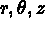; 3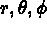.

Array NOP3:
Eventual pointer.

If NBEGM (array NOP2) is non-zero, this integer array contains information which is relative to each super-element or description (this array is not used currently).

Array NOP4:
Vertex coordinates.

This NTYCOO type array contains the NDIM.NP vertex coordinates: X1, Y1, X2, Y2, ..., or X1, Y1, Z1, X2, ...

Array NOP5:
Sequential description of the elements.

This integer array describes each element in the mesh sequentially. It contains:

Loop I from 1 to NE

• NCGE, the element geometry code (1 = node, 2 = segment, 3 = triangle, 4 = quadrilateral, 5 = tetrahedron, 6 = pentahedron, 7 = hexahedron, 8 = super-element, etc.)
• NMAE, the number of words required to store the reference numbers of the faces, edges and vertices,
• NDSDE, the element sub-domain number,
• NNO, the number of nodes in the element,

Loop J from 1 to NNO

• NONO(J), the number of node J of the element,
End of loop J.
• if NCOPNP (see array NOP2) is zero:
• NPO, the number of points in the element, Loop J from 1 to NPO
1. NOPO(J), the number of point J of the element,
End of loop J.
• if NMAE is non-zero:
• INING, level indicator for information regarding the reference numbers.

If INING = 3 only the vertex reference numbers are given, for INING = 2 the edge, followed by the vertex reference numbers are given, and for
INING = 1 the face, edge and vertex reference numbers are specified.

Loop from 2 to NMAE

1. if INING = 1, the face, followed by the edge and vertex, references.
2. if INING = 2, the edge, followed by the vertex, references,
3. if INING = 3, the vertex references,
End of loop.
End of loop I.

INFOGE:
I.D.S. GEOM

Aim:
Print certain items contained in a   D.S. GEOM as a function of selection criteria.

Calling the module:
Via preprocessor INFOXX, or a classical call:

Dimension array M (in the common blank), call INITI, restore the D.S. GEOM to be processed into main memory (see SDREST [George et al. 1989d]) in order to determine the addresses of the generic arrays, GEO2, GEO3, ... GEO7, call the module:

CALL INFOGE (M,IOPT,TYPESD,NIVEAU,TAB2,TAB3,TAB4,
. TAB5,TAB6,TAB7,NUMREC,XREC,YREC,ZREC)

where

• M: the super array
• IOPT: selection option for printing items (see INFONO which proposes the same choices); here we may have:
• 0: nothing
• -1: call IMGEOM where NUMREC is the print parameter
• 1: print array GEO2
• 6: print point numbers with reference NUMREC
• 10: print the elements of sub-domain number NUMREC
• 11: print the coordinates of point number NUMREC
• TYPESD: the type of D.S., here 'GEOM'
• NIVEAU: the level of the D.S.
• TAB2,...,TAB7: arrays 'GEO2'...'GEO7'
• NUMREC: an integer parameter used in the selection process (see IOPT)
• XREC,YREC,ZREC: 3 real parameter values used in the selection process (see IOPT)

Remark:
As a result of the storage method used in this D.S., the choice governed by parameter IOPT may induce delicate and long computations. Consequently, only certain selections are possible. To access other options, we could:

• convert the D.S. GEOM into a D.S. NOPO
• and employ INFONO or INFOXX using this D.S. NOPO.

INFONO:
I.D.S. NOPO

Aim:
Print certain items contained in a  D.S. NOPO as a function of selection criteria.

Calling the module:
Via preprocessor INFOXX, or a classical call:

Dimension array M (in the blank common), call INITI, restore the appropriate D.S. NOPO into main memory (see SDREST [George et al. 1989d]) in order to determine the addresses of the generic arrays NOP2, NOP4 and NOP5, call the module:

CALL INFONO (M,IOPT,TYPESD,NIVEAU,TAB2,TAB4,
. TAB5,NUMREC,XREC,YREC,ZREC)

where

• M: the super array
• IOPT: selection option for items to be printed
• 0: nothing
• -1: call IMNOPO with NUMREC as print parameter
• -2: mesh element verification (surface and volume test)
• 1: print array NOP2
• 2: print element number NUMREC
• 3: print elements containing point NUMREC
• 4: print the elements containing the point coordinates XREC, YREC, ZREC, or nearest
• 5: print the elements containing node NUMREC
• 6: print the numbers of the points with reference NUMREC
• 7: the same as above, but for nodes
• 8: print the elements that have an edge with reference NUMREC
• 9: print the elements that have an edge or face with reference NUMREC
• 10: print the elements with sub-domain number NUMREC
• 11: print the coordinates of point number NUMREC
• 12: print the elements that have a face with reference NUMREC
• TYPESD: the type of D.S., here "NOPO"
• NIVEAU: the D.S. level
• TAB2,...,TAB5: the arrays "NOP2", ..., "NOP5"
• NUMREC: an integer used in the selection process (see IOPT)
• XREC,YREC,ZREC: 3 real numbers used in the selection process (see IOPT)

INITI:

Aim:
Initialize the variables used in all the MODULEF library modules, in particular the initialization   of the dynamic storage. A call to this utility is therefore indispensable in all the main programs written by the user.

Calling the utility:

CALL INITI (M,LM,IMPRE,NNN)

where

• M: the super array
• LM: the number of words in this array
• IMPRE: the output parameter for execution (0: no printout, ..., 10: max printout)
• NNN: the print parameter for arrays (0: no printout, ..., 3 max printout), i.e. each operation concerned with dynamical storage of an array in the super array M is indicated.

Data:
This subroutine reads a TITLE in free format.

Remark:
The subroutine INITIS  has the same arguments as INITI  and fulfills the same role, but does not require a title.

MODNOP:
I.D.S. NOPO
O.D.S. NOPO

Aim:
Construct a mesh  from an existing mesh (I.D.S. NOPO) by translation, anisotropic dilatation, rotation, symmetry, arbitrary linear transformation , or a combination of these transformations.

This method applies to P1 Lagrange type elements.

Calling the module:
Keywords TRAN, DILA, ROTA and SYMD of
 APNOPO (2D) or APNOP3 (3D), or using preprocessor MODNXX, or a classical call:

Dimension array M (in the blank common), declare the integer arrays NNF and NNDSD of length 2 (if not used) or 2 LNNF and 2 LNDSD (see below), declare the integer array ITYPE of length NTRANS, declare the real array TRANS with dimension 12 NTRANS, call INITI:

CALL MODNOP (M,NFNOPE,NINOPE,NFNOPS,NINOPS,NTRANS,
. ITYPE,TRANS,INVER,LNNF,NNF,LNDSD,NNDSD)

where

• M: the super array
• NF(NI)NOPE: the support number of the I.D.S. NOPO and its level
• NF(NI)NOPS: the same for the O.D.S. NOPO
• NTRANS: the number of successive transformations to apply to the mesh
• ITYPE(1:NTRANS): the type of NTRANS transformations:
• 0: identity
• 1: translation of vector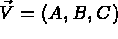• 2: symmetry with respect to a line with equation Ax+By+C=0, or a plane with equation Ax+By+Cz+D=0
• 3: rotation by angle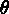around a point (2D), or an axis (3D)
• 4: anisotropic dilatation with coefficients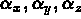• 10: arbitrary linear transformation defined explicitly
• TRANS(1:12,1:NTRANS): the values defining the transformations with, for transformation I:
• identity: nothing
• translation: TRANS(1,I)=A, TRANS(2,I)=B and TRANS(3,I)=C the vector components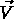• 2D symmetry: TRANS(1,I)=A, TRANS(2,I)=B and TRANS(3,I)=C the parameters defining the symmetry
• 3D symmetry: TRANS(1,I)=A, TRANS(2,I)=B, TRANS(3,I)=C and TRANS(4,I)=D the parameters defining the symmetry
• 2D rotation: TRANS(4,I)=X0, TRANS(5,I)=Y0, the center of rotation and TRANS(7,I)=, its angle in degrees
• 3D rotation: TRANS(1,I)=A, TRANS(2,I)=B, TRANS(3,I)=C, the components of the rotational axis and TRANS(4,I)=X0,
 TRANS(5,I)=Y0, TRANS(6,I)=Z0, TRANS(7,I)=, the coordinates of an invariant point and the rotation angle in degrees
• 2D dilatation: TRANS(1,I)=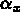, TRANS(2,I)=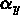, the dilation coefficients and TRANS(4,I)=X0, TRANS(5,I)=Y0, an invariant point
• 3D dilatation: TRANS(1,I)=, TRANS(2,I)=, TRANS(3,I)=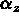, the dilation coefficients and TRANS(4,I)=X0, TRANS(5,I)=Y0,
TRANS(6,I)=Z0, the coordinates of an invariant point
• linear transformation: A(1:3,1:3) and B(1:3), the matrix and RHS vector of the transformation defined by X' = AX + B.
The 9 terms of the matrix are input line per line in TRANS(1,I) to TRANS(9,I), the 3 terms of B are input in TRANS(10,I), ...
• INVER: element inversion if 1, 0 if not (see remark below)
• LNNF: the number of references to modify
• NNF: the reference correspondence array:
If= NNF(1,j) and= NNF(2,j) then the items with number(j-th number to modify) will have the numberin the O.D.S.
• LNDSD: the number of sub-domain numbers to modify
• NNF: the array containing the correspondence between these numbers (same logic)

Remarks:

In the case of a negative isometry, it is necessary to inverse the direction of all the elements. This is left to the user: we will have inversion for the case where we perform a symmetry, a dilatation with 1 or 3 negative coefficients and for all linear transformations corresponding to a negative isometry.

The transformed mesh can be equipped with other physical attributes by associating new values to old reference or sub-domain numbers.

This module can be used to change the physical numbers of the mesh by defining an identity as a geometric transformation.

NOP2P1:
I.D.S. NOPO
O.D.S. NOPO

Aim:
Transforms an I.D.S. NOPO , containing P2 type triangles, into an O.D.S. NOPO with P1 type triangles. Each P2 element is split into 3 P1 triangles. The node numbering remains unchanged.

This module permits post-processing. In fact, if a problem exhibits symmetries, it could be solved on one part of the domain only. It is, on the other hand, interesting to plot the results on the entire domain. This is done by creating the symmetrical mesh of the part treated and the solution obtained by symmetry from the solution actually calculated; the 2 meshes and 2 solutions obtained must then be added together (see RECOLC). These type of operations are only possible for P1 (or Q1) meshes. The initial data must thus be converted to P1 (or Q1), which is the aim of this module.

Calling the module:
Classical call:

Dimension array M (in the blank common), call INITI

CALL NOP2P1 (M,NFNOPE,NINOPE,NFNOPS,NINOPS)

where

• M: is the super array
• NF(NI)NOPE: the support number of the I.D.S. NOPO and its level
• NF(NI)NOPS: the same for the O.D.S. NOPO.

NOPGEO:
I.D.S. NOPO
O.D.S. GEOM

Aim:
Convert  an I.D.S. of type NOPO  into a O.D.S. of type GEOM.
Calling the module:
Via preprocessor NOPGXX, or a classical call:

Dimension array m (in the blank common), call INITI

CALL NOPGEO (M,NFNOPO,NINOPO,NFGEOM,NIGEOM)

where

• M: is the super array
• NF(NI)NOPO: the support number of the I.D.S. NOPO and its level
• NF(NI)GEOM: the same for the O.D.S. GEOM.

QUA4TR:
I.D.S. NOPO
O.D.S. NOPO
Aim:
Transform a  I.D.S. NOPO containing triangles and quadrilaterals into a O.D.S. NOPO containing only triangles. Each quadrilateral element is split  into 4 triangles.
Calling the module:
Keyword Q4T of APNOPO, or a classical call:

Dimension array M (in the blank common), call INITI

CALL QUA4TR (M,NFNOPE,NINOPE,NFNOPS,NINOPS)

where

• M: is the super array
• NF(NI)NOPE: the support number of the I.D.S. NOPO and its level
• NF(NI)NOPS: the same for the O.D.S. NOPO.

I.D.S. NOPO
O.D.S. NOPO
Aim:
create a mesh consisting of quadrangles from mesh   consisting of triangles (some triangles can be kept in the mesh)
Calling the module:
Classical call

Dimension array M (in the blank common), call INITI

where

• M: is the super array
• NF(NI)NOPE: the support number of the I.D.S. NOPO and its level
• NF(NI)NOPS: the same for the O.D.S. NOPO.

QUALNO:
I.D.S. NOPO

Aim:
measure   the quality of mesh. See [Modulef User Guide -6]
Calling the module:
Via the preprocessor QUALXX

RECOLC:
I.D.S. NOPO and B
O.D.S. NOPO and B
Aim:
Add  together 2 meshes (the 2 I.D.S. NOPO) in order to form one single mesh (O.D.S. NOPO).

Furthermore, this module can be used to add  2 D.S. B, containing the solution corresponding to the 2 initial meshes in order to form the D.S. B corresponding to the mesh resulting from pasting together the 2 initial meshes.

Meshes and solutions are of type P1.

The method used to allocate the common items (points, edges and faces) in the 2 initial meshes is based on encoding the point positions by a hashing process. The common points are of two types:

• geometrical identity: 2 points are said to be identical if they have the same coordinates,
• total identity: 2 points are said to be totally identical if their positions are the same and if, furthermore, their reference numbers are the same.

The edges and faces are said to be identical if their extremities (in terms of points) are the same; we also have the distinction seen above where the reference number is either taken into account, or not, in the item under consideration.

The parameterdefines the precision of the "gluing" operation.

Calling the module:
Keyword RECO of APNOPO or APNOP3, or via preprocessor RECOXX, or by a classical call:

Dimension array M (in the blank common), call INITI

CALL RECOLC (M,M,EPS,NFNOP1,NINOP1,NFNOP2,NINOP2,
. IOPT,IEB,NFB1,NIB1,NFB2,NIB2,NFNOPS,NINOPS,NTNOPS,
. NFBS,NIBS,NTBS)

where

• M: the super array
• EPS: the precision of the "gluing" together of the meshes
• NF(NI)NOP1: the support number of the I.D.S. NOPO number 1 and its level
• NF(NI)NOP2: the support number of the I.D.S. NOPO number 2 and its level
• IOPT: "gluing" option
• 0: geometric "gluing"
• 1: total "gluing", i.e. taking the physical attributes into account
• IEB: "gluing" together of 2 meshes if 0, of 2 meshes and their solutions if 1
• NF(NI)B1: the support number of the I.D.S. B number 1 and its level
• NF(NI)B2: the support number of the I.D.S. B number 2 and its level
• NF(NI)NOPS: the support number of the O.D.S. NOPO and its level
• NF(NI)BS: the support number of the O.D.S. B and its level
• NT(NOPS)BS: the number of arrays associated to the O.D.S. (generally 0)

Remarks:

In order to "glue" together large meshes, two super arrays, M1 and M2, could be used and the module can then be called by
 CALL RECOLC(M1,M2,...)

If NTNOPS or NTBS is non-zero, it is necessary to input the following information on data cards (free format):

Loop I from 1 to NTNOPS (NTBS)

• name of array I in 4 characters
• type of array (1 = integer, 2 = real simple precision, 4 = character, 5 = real double precision, ...)
• number of words in this array
• the variables of this array
• comments on array I in 18 words of 4 characters.
End of loop.

REFNOP:
I.D.S. NOPO

Aim:
Find the elements of a face referenced by a given number (3D case), or an edge referenced by a given number (2D case), in a mesh .

The element numbers are output to an array for later use.

Calling the module:
Via preprocessor REFNXX, or a classical call:

Dimension array M (in the blank common), declare the integer array, TABNUM, of LTAB words, call INITI

CALL REFNOP (M,M,M,NFNOPE,NINOPE,NREF,TABNUM,LONG)

where

• M: the super array
• NF(NI)NOPE: the support number of the I.D.S. NOPO and its level
• NREF: the reference number sought
• TABNUM [O]: the numbers of the elements located
• LONG [O]: the total number of these elements

Remark:
On entry, LTAB is set to a maximum as LONG is only known after computation.

REFPOI:
I.D.S. NOPO

Aim:
Find the points with reference numbers given in a mesh .

Output the numbers of these points to an array for later use.

Calling the module:
Via preprocessor REFPXX, or a classical call:

Dimension array M (in the blank common), declare the integer array, TABNUM, of LTAB words long, call INITI

CALL REFPOI (M,M,M,IACOOR,NP,NFNOPE,NINOPE,NREF,
. TABNUM,LONG)

where

• M: the super array
• NF(NI)NOPE: the support number of the I.D.S. NOPO and its level
• NREF: the reference number sought
• TABNUM [O]: the numbers of the points located
• LONG [O]: the total number of these points
• IACOOR [O]: address in M of array NOP4
• NP [O]: number of points in the mesh

Remark:
On entry, LTAB is set to a maximum as LONG is only known after computation.

REGMA2:
I.D.S. NOPO
O.D.S. NOPO

Aim:
Regularize a 2D mesh  by displacing each non-boundary vertex or vertex not specified by barycentering .

Certain points are not affected by this transformation:

• the points situated on the boundary of the domain
• all points belonging to 2 elements with different sub-domain numbers
• all points specified by the user
• all non-zero reference points.

Calling the module:
Keyword REGU of APNOPO, or a classical call:

Dimension array M (in the blank common), declare the integer array, NPFIX, of NBFIX words, call INITI

CALL REGMA2 (M,NFNOPE,NINOPE,NFNOPS,NINOPS,
. NBI,NPFIX,NBFIX)

where

• M: the super array
• NF(NI)NOPE: the support number of the I.D.S. NOPO and its level
• NF(NI)NOPS: the support number of the O.D.S. NOPO and its level
• NBI: the number of iterations to perform
• NPFIX: the set of points fixed by the user
• NBFIX: the total number of fixed points

inter.psfig:regu2Initial meshinterregu.psfig:regu21Regularized

RETRIN:
I.D.S. NOPO
O.D.S. NOPO

Aim:
Split  the  elements of a mesh into sub-elements of the same type.
Calling the module:
Keyword RETR of APNOPO or APNOP3, or via preprocessor RETRXX, or by a classical call:

Dimension array M (in the blank common), declare the integer array NNFRON of 1 or NBFRON words, declare the logical FONINT and the external FFRONT, call INITI

CALL RETRIN (M,NFNOPE,NINOPE,NFNOPS,NINOPS,NAUXI,N,
. NBFRON,NNFRON,FONINT,FFRONT)

where

• M: the super array
• NF(NI)NOPE: the support number of the I.D.S. NOPO and its level
• NF(NI)NOPS: the support number of the O.D.S. NOPO and its level
• NAUXI: not used
• N: the number of subdivisions, each element resulting in N� or N� elements per splitting. If N=-1 and for a mesh composed of triangles only, each triangle is split into three sub-triangles using the barycentre.
• NBFRON: the number of reference numbers defining the curved boundaries (2D case), or 0 (3D case)
• NNFRON: the set of reference numbers to describe in function
 FFRONT
• FONINT: initialized to .FALSE.
• FFRONT: the name of the subroutine used for eventual projections

Remark:
Function FFRONT is described in chapter 1.

afffin.psfig:retrInitial meshretr.psfig:retr1After splitting

ROTNOP:
I.D.S. NOPO
O.D.S. NOPO

Aim:
Deform  a mesh (I.D.S. NOPO) by rotation .

The method applies to P1 Lagrange type elements.

Calling the module:
Keyword ROTA of APNOPO (2D), or APNOP3 (3D), or a classical call:

Dimension array M (in the blank common), declare the integer arrays, NNF and NNDSD, of length 2 (if not used), or 2 LNNF and 2 LNDSD (see below), call INITI

CALL ROTNOP (M,NFNOPE,NINOPE,NFNOPS,NINOPS,A,B,C,X0,
. Y0,Z0,TETA,LNNF,NNF,LNDSD,NNDSD)

where

• M: the super array
• NF(NI)NOPE: the support number of the I.D.S. NOPO and its level
• NF(NI)NOPS: the same for the O.D.S. NOPO
• A,B,C: the components of the rotational axis
• X0,Y0,Z0: the coordinates of a point on this axis, center of rotation
• TETA: the rotational angle,, in degrees
• LNNF: the number of references to modify
• NNF: the array signifying the correspondence between the references:
If= NNF(1,j) and= NNF(2,j) then the items with number(j-th number to modify) will have the numberin the O.D.S.
• LNDSD: the number of sub-domain numbers to modify
• NNF: the array containing the correspondence between these numbers (same logic as above)

Remark:
In 2D, A, B, C and Z0 are not used.

SYMNOP:
I.D.S. NOPO
O.D.S. NOPO

Aim:
Deform  a mesh (I.D.S. NOPO) by symmetry .

The method applies to P1 Lagrange type elements.

Calling the module:
Keyword SYMD of APNOPO (2D), or SYMP of APNOP3 (3D), or a classical call:

Dimension array M (in the blank common), declare the integer arrays, NNF and NNDSD, of length 2 (if not used), or 2 LNNF and 2 LNDSD (see below), call INITI

CALL SYMNOP (M,NFNOPE,NINOPE,NFNOPS,NINOPS,A,B,C,D,
. LNNF,NNF,LNDSD,NNDSD)

where

• M: the super array
• NF(NI)NOPE: the support number of the I.D.S. NOPO and its level
• NF(NI)NOPS: the same for the O.D.S. NOPO
• A,B,C,D: the coefficients of the equation of the line or plane of symmetry
• LNNF: the number of references to modify
• NNF: the array containing the correspondence between the references:
If= NNF(1,j) and= NNF(2,j) then the items with number(j-th number to modify) will have the numberin the O.D.S.
• LNDSD: the number of sub-domain numbers to modify
• NNF: the array containing the correspondence between these numbers (same logic as above)

Remark:
In 2D, D is not used.

TN3D2D:
I.D.S. NOPO
O.D.S. NOPO

Aim:
Transform a  3D mesh (I.D.S. NOPO) into a 2D mesh by setting the z-components to zero.

The method applies to P1 Lagrange type elements.

Calling the module:
Classical call:

Dimension array M (in the blank common), call INITI

CALL TN3D2D (M,NFNOPE,NINOPE,NFNOPS,NINOPS)

where

• M: the super array
• NF(NI)NOPE: the support number of the I.D.S. and its level
• NF(NI)NOPS: the same for the O.D.S. NOPO

Remark:
No verification is performed.

TRANOP:
I.D.S. NOPO
O.D.S. NOPO

Aim:
Deform a mesh  (I.D.S. NOPO) by translation .

The method applies to P1 Lagrange type elements.

Calling the module:
Keyword TRAN of APNOPO (2D) or APNOP3 (3D), or a classical call:

Dimension array M (in the blank common), declare the integer arrays, NNF and NNDSD, of length 2 (if not used), or 2 LNNF and 2 LNDSD (see below), call INITI

CALL TRANOP (M,NFNOPE,NINOPE,NFNOPS,NINOPS,A,B,C,
. LNNF,NNF,LNDSD,NNDSD)

where

• M: the super array
• NF(NI)NOPE: the support number of the I.D.S. NOPO and its level
• NF(NI)NOPS: the same for the O.D.S. NOPO
• A,B,C: the coefficients of the translation vector
• LNNF: the number of references to modify
• NNF: the array containing the correspondence between the references:
If= NNF(1,j) and= NNF(2,j) then the items with number(j-th number to modify) will have the numberin the O.D.S.
• LNDSD: the number of sub-domain numbers to modify
• NNF: the array containing the correspondence between these numbers (same logic as above)

Remark:
In 2D, C is not used.

TRCOAC:
I.D.S. NOPO
O.D.S. NOPO

Aim:
Construct a mesh  (O.D.S. NOPO) containing the faces (3D) or edges (2D) in the initial mesh, with given references numbers, as elements.

Calling the module:
keyword EXTR of APNOPO (2D) or APNOP3 (3D), or a classical call:

Dimension array M (in the blank common), declare the integer array, REF, of length NBREF, call INITI

CALL TRCOAC (M,M,M,REF,NBREF,NFNOPE,NINOPE,NFNOPS,
. NINOPS)

where

• M: is the super array
• REF: the set of reference numbers to consider
• NBREF: the total number of reference numbers to extract
• NF(NI)NOPE: the support number of the I.D.S. NOPO and its level
• NF(NI)NOPS: the same for the O.D.S NOPO

TRNOPO:
I.D.S. NOPO or GEOM

Aim:
Plot  a 2D or 3D mesh  (I.D.S. NOPO or I.D.S. GEOM).
Calling the module:
Keyword DESS of APNOPO (2D) or APNOP3 (3D) (see the remark relating to this keyword), or via preprocessor TRNOXX .

For more detail, consult the MODULEF report [George et al. 1987].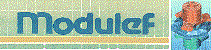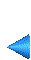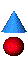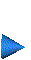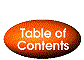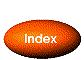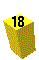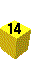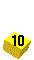Next: 5 Tutorial examples Up: 4 List of the modules Prev: 4.2 Mesh generation modules Index Contents